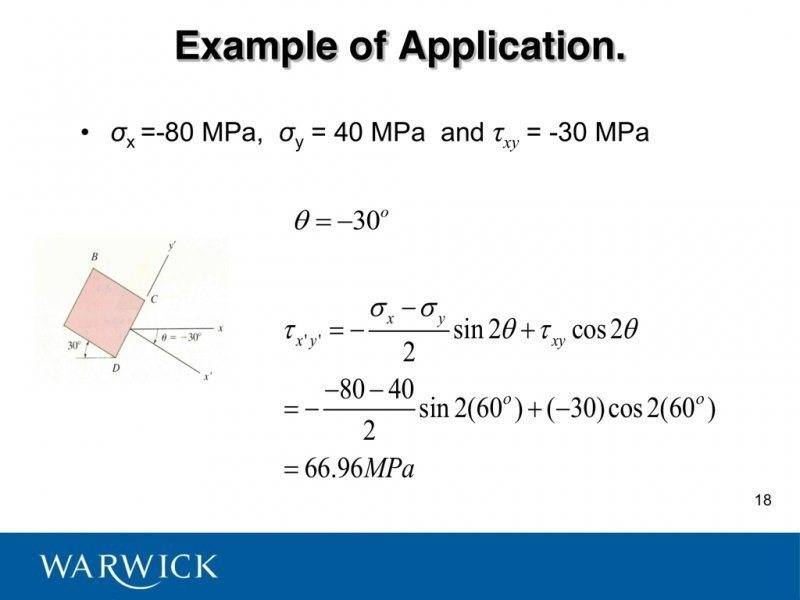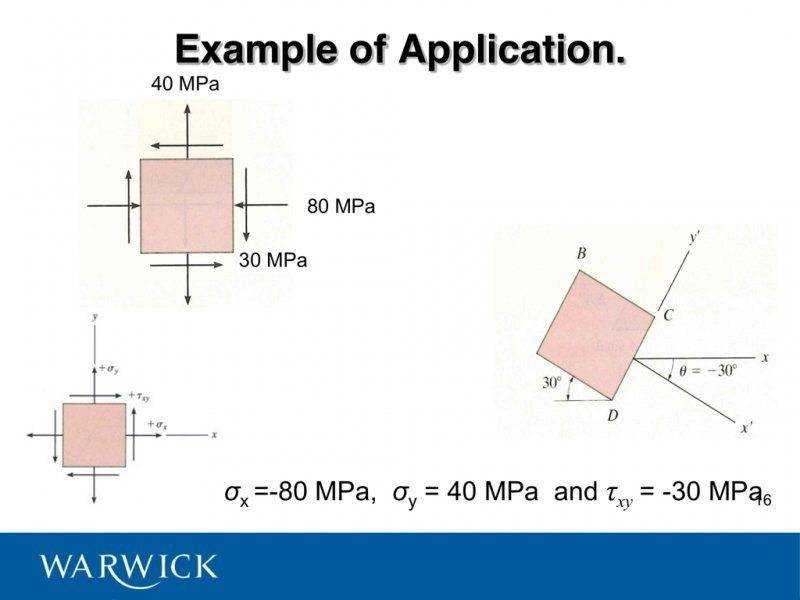# Is this a mistake? (Stress Plane transformations)This is a screenshot from my lecture notes provided by my lecturer. He says theta=-30 and then proceeds to use a value of theta=60? Surely this is wrong?Chestermiller
Mentor
Did you not notice the 2θ in the equation?

Did you not notice the 2θ in the equation?

I did but θ = -30 so surely he should have used Sin[2(-30)] rather than Sin[2(60)] as he's done above?

Chestermiller
Mentor
I did but θ = -30 so surely he should have used Sin[2(-30)] rather than Sin[2(60)] as he's done above?
You're right!!! And that's not the only error there. They did evaluate the sin and cos of 60 correctly in their equation (omitting the factor of 2), but the second term should have been subtracted, rather than added (as they did). The answer should be 36.96, not 66.96.

Chet